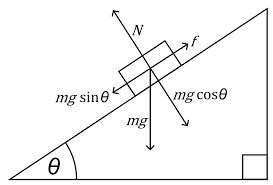## 2014-09-10

### Forces in Equilibrium II

As we have learnt, when forces are in a state of equilibrium, the net force (or also known as resultant force) is zero. The object is either at rest or in a motion with constant velocity (hence zero acceleration).

Examples of two forces in equilibrium are a plate resting on a table or a skydiver falling at a constant velocity which is also known as terminal velocity.

An object that  is resting on a tilted or inclined plane also can be in equilibrium with each other. In this case three forces are in equilibrium.N is the normal reaction.
Weight is represented by mg where m = mass of the object and g is the gravitational constant.

Addition of forces and Resultant Force

In this example, you can see that there are three different ways on how a 40N force and a 30N force can react. In the first example both forces react in the same direction.

In the second example, both forces react in opposite direction. Hence the resultant force will be 10N in the direction of the greater force (40N).

In the third example, The two forces are in perpendicular with each other. If you are using the parallelogram method ( Or Phythagoras theorem!) you can easily find that the resultant force is 50N.So if it helps, resultant forces can be categorised in three situations:

1. Two parallel forces
2. Two non-parallel forces
3. Two perpendicular forces

at GCE-O level, you may be asked to find the resultant force for only two forces. But in reality or more complex situations there are many forces that act on an object.